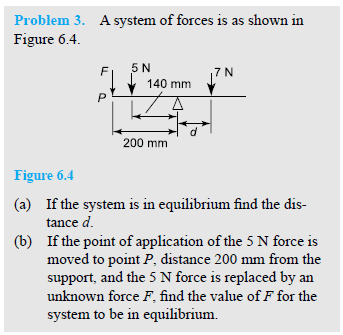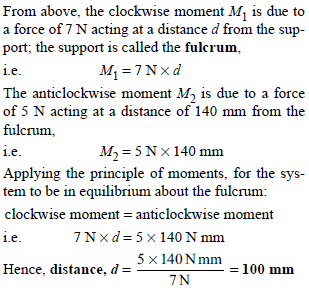# Equilibrium and principle of moments

## Homework Statement## Homework Equations

Torque = F x D
Manticlockwise = Mclockwise

## The Attempt at a Solution

From what I understand, the moment on left hand side of the pivot point needs to be equal to the moment on right hand side, therefore...

(Fx.2)+(5x.14) = (7xd)

My initial thinking was to substitute 2N into F, so that both forces on either side of pivot point are equal to 7N. However, this was not correct because the resultant forces were not in equilibrium after checking.

[/B]Could someone explain to me why F wasn't factored into the working? The way I'm interpreting this is that there is no force acting at point F. Would this be a correct assumption?

CWatters
Homework Helper
Gold Member
Looks like a badly worded question to me.

If you read Question (b) it says F replaces the 5N force. So F doesn't exist for question (a) ?

CWatters
Homework Helper
Gold Member
(Fx.2)+(5x.14) = (7xd)

If F does exist for (a) then that's correct but you can't solve it. You have one equation and two unknowns so best you can do is write an equation for d in terms of F.

NascentOxygen
Staff Emeritus